International
Tables for
Crystallography
Volume H
Powder diffraction
Edited by C. J. Gilmore, J. A. Kaduk and H. Schenk

International Tables for Crystallography (2018). Vol. H, ch. 3.8, pp. 327-329

## Section 3.8.3. Cluster analysis

C. J. Gilmore,a G. Barra and W. Donga*

aDepartment of Chemistry, University of Glasgow, University Avenue, Glasgow, G12 8QQ, UK
Correspondence e-mail:  chris@chem.gla.ac.uk

### 3.8.3. Cluster analysis

| top | pdf |

Cluster analysis uses d (or s, or δ) to partition the patterns into groups based on the similarity of their diffraction profiles. Associated with cluster are a number of important ancillary techniques all of which will be discussed here. A flowchart of these methods is shown in Fig. 3.8.4.

#### 3.8.3.1. Dendrograms

| top | pdf |

Using d and s, agglomerative, hierarchical cluster analysis is now carried out, in which the patterns are put into clusters as defined by their distances from each other. [Gordon (1981, 1999) and Everitt et al. (2001) provide excellent and detailed introductions to the subject. Note that the two editions of Gordon's monograph are quite distinct and complementary.] The method begins with a situation in which each pattern is considered to be in a separate cluster. It then searches for the two patterns with the shortest distance between then, and joins them into a single cluster. This continues in a stepwise fashion until all the patterns form a single cluster. When two clusters (Ci and Cj) are merged, there is the problem of defining the distance between the newly formed cluster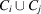and any other cluster Ck. There are a number of different ways of doing this, and each one gives rise to a different clustering of the patterns, although often the difference can be quite small. A general algorithm has been proposed by Lance & Williams (1967), and is summarized in a simplified form by Gordon (1981). The distance from the new cluster formed by merging Ci and Cj to any other cluster Ck is given by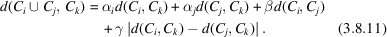There are many possible clustering methods. Table 3.8.1defines six commonly used clustering methods, defined in terms of the parameters α, β and γ. All these methods can be used with powder data; in general, the group-average-link or single-link formalism is the most effective, although differences between the methods are often slight.

 Table 3.8.1| top | pdf | Six commonly used clustering methods
 For each method, the coefficients αi, β and γ in equation (3.8.11)are given.
Methodαiβγ
Single link ½ 0 −½
Complete link ½ 0 ½
Average link ni/(ni + nj) 0 0
Weighted-average link ½ 0 0
Centroid ni/(ni + nj) ninj/(ni + nj)2 0
Sum of squares (ni + nk)/(ni + nj + nk) nk/(ni + nj + nk) 0

The results of cluster analysis are usually displayed as a dendrogram, a typical example of which is shown in Fig. 3.8.6(a), where a set of 13 powder patterns is analysed using the centroid method. Each pattern begins at the bottom of the plot as a separate cluster, and these amalgamate in stepwise fashion linked by horizontal tie bars. The height of the tie bar represents a similarity measure as measured by the relevant distance. As an indication of the differences that can be expected in the various algorithms used for dendrogram generation, Fig. 3.8.6(e) shows the same data analysed using the single-link method: the resulting clustering is slightly different: the similarity measures are larger, and, in consequence, the tie bars are higher on the graph. [For further examples see Barr et al. (2004b,c) and Barr, Dong, Gilmore & Faber (2004).]

#### 3.8.3.2. Estimating the number of clusters

| top | pdf |

An estimate of the number of clusters present in the data set is needed. In terms of the dendrogram, this is equivalent to `cutting the dendrogram' i.e. the placement of a horizontal line across it such that all the clusters as defined by tie lines above this line remain independent and unlinked. The estimation of the number of clusters is an unsolved problem in classification methods. It is easy to see why: the problem depends on how similar the patterns need to be in order to be classed as the same, and how much variability is allowed within a cluster. We use two approaches: (a) eigenvalue analysis of matrices ρ and A, and (b) those based on cluster analysis.

Eigenvalue analysis is a well used technique: the eigenvalues of the relevant matrix are sorted in descending order and when a fixed percentage (typically 95%) of the data variability has been accounted for, the number of eigenvalues is selected. This is shown graphically via a scree plot, an example of which is shown in Fig. 3.8.2.Figure 3.8.2 | top | pdf |Four different methods of estimating the number of clusters present in a set of 23 powder patterns for the drug doxazosin. A total of five polymorphs are present, as well as two mixtures of these polymorphs. (a) A scree plot from the eigenvalue analysis of the correlation matrix; (b) the use of the C test (the coefficients have been multiplied by 100.0), which gives an estimate of five clusters using its local maximum. The γ test estimates that there are seven clusters and the CH test has a local maximum at seven clusters. Numerical details are given in Table 3.8.2.

We carry out eigenvalue analysis on the following:

 (1) Matrix ρ. (2) Matrix A, as described in Section 3.8.3.3. (3) A transformed form of ρ in which ρ is standardized to give ρs in which the rows and columns have zero mean and unit variance. The matrix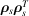is then computed and subjected to eigenanalysis. It tends to give a lower estimate of cluster numbers than (1).

The most detailed study on cluster counting is that of Milligan & Cooper (1985), and is summarized by Gordon (1999). From this we have selected three tests that seem to operate effectively with powder data:

 (4) The Calinški & Harabasz (1974) (CH) test: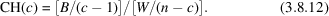A centroid is defined for each cluster. W denotes the total within-cluster sum of squared distances about the cluster centroids, and B is the total between-cluster sum of squared distances. Parameter c is the number of clusters chosen to maximize CH. (5) A variant of Goodman & Kruskal's (1954) γ test, as described by Gordon (1999). The dissimilarity matrix is used. A comparison is made between all the within-cluster dissimilarities and all the between-cluster dissimilarities. Such a comparison is marked as concordant if the within-cluster dissimilarity is less than the between-cluster dissimilarity, and discrepant otherwise. Equalities, which are unusual, are disregarded. If S+ is the number of concordant and S− the number of discrepant comparisons, then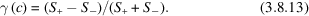A maximum in γ is sought by an appropriate choice of cluster numbers. (6) The C test (Milligan & Cooper, 1985). This chooses the value of c that minimizes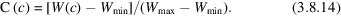W(c) is the sum of all the within-cluster dissimilarities. If the partition has a total of r such dissimilarities, then Wmin is the sum of the r smallest dissimilarities and Wmax is the sum of the r largest.

The results of tests (4)–(6) depend on the clustering method being used. To reduce the bias towards a given dendrogram method, these tests are carried out on four different clustering methods: the single-link, the group-average, the sum-of-squares and the complete-link methods. Thus there are 12 semi-independent estimates of the number of clusters from clustering methods, and three from eigenanalysis, making 15 in all.

A composite algorithm is used to combine these estimates. The maximum and minimum values of the number of clusters (cmax and cmin, respectively) given by the eigenanalysis results [(1)–(3) above] define the primary search range; tests (4)–(6) are then used in the range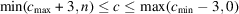to find local maxima or minima as appropriate. The results are averaged, any outliers are removed, and a weighted mean value is taken of the remaining indicators, then this is used as the final estimate of the number of clusters. Confidence levels for c are also defined by the estimates of the maximum and minimum cluster numbers after any outliers have been removed.

A typical set of results for the PXRD data from 23 powder patterns for doxazosin (an anti-hypertension drug) in which five polymorphs are present, as well as two mixtures of polymorphs, is shown in Fig. 3.8.2(a) and (b) (see also Table 3.8.2). The scree plot arising from the eigenanalysis of the correlation matrix indicates that 95% of the variability can be accounted for by five components, and this is shown in Fig. 3.8.2(a). Eigenvalues from other matrices indicate that four clusters are appropriate. A search for local optima in the CH, γ and C tests is then initiated in the range 2–8 possible clusters. Four different clustering methods are tried, and the results indicate a range of 4–7 clusters. There are no outliers, and the final weighted mean value of 5 is calculated. As Fig. 3.8.2(b) shows, the optimum points for the C and γ tests are often quite weakly defined (Barr et al., 2004b).

 Table 3.8.2| top | pdf | Estimate of the number of clusters for the 23 sample data set for doxazosin
 There are five polymorphs present, plus two mixtures of these polymorphs. The maximum estimate is 7; the minimum estimate is 4; the combined weighted estimate of the number of clusters is 6, and the median value is 5. The dendrogram cut level is set to give 5 clusters, and the lower and upper confidence limits are 4 and 7, respectively.
MethodNo. of clusters
Principal-component analysis (non-transformed matrix) 5
Principal-component analysis (transformed matrix) 4
Multidimensional metric scaling 4
γ statistic using single linkage 7
CH statistic using single linkage 7
C statistic using single linkage
γ statistic using group averages 7
CH statistic using group averages 5
C statistic using group averages
γ statistic using sum of squares
CH statistic using sum of squares 5
C statistic using sum of squares
γ statistic using complete linkage
CH statistic using complete linkage 5
C statistic using complete linkage

#### 3.8.3.3. Metric multidimensional scaling

| top | pdf |

This is, in its essentials, the particle-in-a-box problem. Each powder pattern is represented as a single sphere, and these spheres are placed in a cubic box of unit dimensions such that the positions of the spheres reproduce as closely as possible the distance matrix, d, generated from correlating the patterns. The spheres have an arbitrary orientation in the box.

To do this, the (n × n) distance matrix d is used in conjunction with metric multidimensional scaling (MMDS) to define a set of p underlying dimensions that yield a Euclidean distance matrix, dcalc, whose elements are equivalent to or closely approximate the elements of d.

The method works as follows (Cox & Cox, 2000; Gower, 1966; Gower & Dijksterhuis, 2004).

The matrix d has zero diagonal elements, and so is not positive semidefinite. A positive definite matrix, A(n × n) can be constructed, however, by computing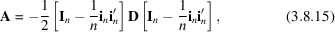where In is an (n × n) identity matrix, in is an (n × 1) vector of unities and D is defined in equation (3.8.8). The matrix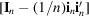is called a centring matrix, since A has been derived from D by centring the rows and columns.

The eigenvectors v1, v2,…, vn and the corresponding eigenvalues λ1, λ2,…, λn are then obtained. A total of p eigenvalues of A are positive and the remaining (np) will be zero. For the p non-zero eigenvalues a set of coordinates can be defined via the matrix X(n × p),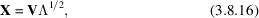where Λ is the vector of eigenvalues.

If p = 3, then we are working in three dimensions, and the X(n × 3) matrix can be used to plot each pattern as a single point in a 3D graph. This assumes that the dimensionality of the problem can be reduced in this way while still retaining the essential features of the data. As a check, a distance matrix dcalc can be calculated from X(n × 3) and correlated with the observed matrix d using both the Pearson and Spearman correlation coefficients. In general the MMDS method works well, and correlation coefficients greater than 0.95 are common. For large data sets this can reduce to ∼0.7, which is still sufficiently high to suggest the viability of the procedure. Parallel coordinates based on the MMDS analysis can also be used, and this is discussed in Sections 3.8.4.2.1and 3.8.4.2.2.

There are occasions in which the underlying dimensionality of the data is 1 or 2, and in these circumstances the data project onto a plane or a line in an obvious way without any problems.

An example of an MMDS plot is shown in Fig. 3.8.6(b), which is linked to the dendrogram in Fig. 3.8.6(a).

#### 3.8.3.4. Principal-component analysis

| top | pdf |

It is also possible to carry out principal-component analysis (PCA) on the correlation matrix. The eigenvalues of the correlation matrix can be used to estimate the number of clusters present via a scree plot, as shown in Fig. 3.8.2(a), and the eigenvectors can be used to generate a score plot, which is an X(n × 3) matrix and can be used as a visualization tool in exactly the same way as the MMDS method to indicate which patterns belong to which class. Score plots traditionally use two components with the data thus projected on to a plane; we use 3D plots in which three components are represented. In general, we find that the MMDS representation of the data is nearly always superior to the PCA analysis for powder and spectroscopic data.

#### 3.8.3.5. Choice of clustering method

| top | pdf |

It is possible to use the MMDS plot (or, alternatively, PCA score plots) to assist in the choice of clustering method, since the two methods operate semi-independently. The philosophy here is to choose a technique that results in the tightest, most isolated clusters as follows:

 (1) The MMDS formalism is used to derive a set of three-dimensional coordinates stored in matrix X(n × 3). (2) The number of clusters, c, is estimated as described in Section 3.8.3.2. (3) Each of six dendrogram methods (see Table 3.8.1) is employed in turn, stopping when c clusters have been generated. Each entry in X can now be assigned to a cluster. (4) A sphere is drawn around each point in X and the average between-cluster overlap of the spheres is calculated for each of the N clusters C1 to CN. If the total number of overlaps is m, this can be written as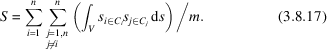If the clusters are well defined then S should be a minimum. Conversely, poorly defined clusters will tend to have large values of S. In the algorithm used in PolySNAP (Barr, Dong & Gilmore, 2009) and DIFFRAC.EVA (Bruker, 2018), the sphere size depends on the number of diffraction patterns. (5) The tightness of each cluster is also estimated by computing the mean within-cluster distance. This should also be a minimum for well defined, tight clusters. (6) The mean within-cluster distance from the centroid of the cluster can also be computed, which should also be a minimum. (7) Steps (4)–(6) are repeated using coordinates derived from PCA 3D score plots. (8) Tests (4)–(7) are combined in a weighted, suitably scaled mean to give an overall figure of merit (FOM); the minimum is used to select which dendrogram method to use (Barr et al., 2004b).

#### 3.8.3.6. The most representative sample

| top | pdf |

Similar techniques can be used to identify the most representative sample in a cluster. This is defined as the sample that has the minimum mean distance from every other sample in the clusters, i.e. for cluster J containing m patterns, the most representative sample, i, is defined as that which gives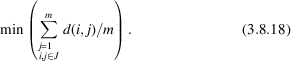The most representative sample is useful in visualization and can, with care, be used to create a database of known phases (Barr et al., 2004b).

#### 3.8.3.7. Amorphous samples

| top | pdf |

Amorphous samples are an inevitable consequence of high-throughput experiments, and need to be handled correctly if they are not to lead to erroneous indications of clustering. To identify amorphous samples the total background for each pattern is estimated and its intensity integrated; the integrated intensity of the non-background signal is then calculated. If the ratio falls below a preset limit (usually 5%, but this may vary with the type of samples under study) the sample is treated as amorphous. The distance matrix is then modified so that each amorphous sample is given a distance and dissimilarity of 1.0 from every other sample, and a correlation coefficient of zero. This automatically excludes the samples from the clustering until the last amalgamation steps, and also limits their effect on the estimation of the number of clusters (Barr et al., 2004b). Of course, the question of amorphous samples is not a binary (yes/no) one: there are usually varying degrees of amorphous content, which further complicates matters.

### References

Barr, G., Dong, W. & Gilmore, C. J. (2004a). High-throughput powder diffraction. II. Applications of clustering methods and multivariate data analysis. J. Appl. Cryst. 37, 243–252.Google Scholar
Barr, G., Dong, W. & Gilmore, C. J. (2004b). High-throughput powder diffraction. IV. Cluster validation using silhouettes and fuzzy clustering. J. Appl. Cryst. 37, 874–882.Google Scholar
Barr, G., Dong, W. & Gilmore, C. J. (2009). PolySNAP3: a computer program for analysing and visualizing high-throughput data from diffraction and spectroscopic sources. J. Appl. Cryst. 42, 965–974.Google Scholar
Barr, G., Dong, W., Gilmore, C. & Faber, J. (2004). High-throughput powder diffraction. III. The application of full-profile pattern matching and multivariate statistical analysis to round-robin-type data sets. J. Appl. Cryst. 37, 635–642.Google Scholar
Bruker (2018). DIFFRAC.EVA: software to evaluate X-ray diffraction data. Version 4.3. https://www.bruker.com/eva .Google Scholar
Calinški, T. & Harabasz, J. (1974). A dendritic method for cluster analysis. Commun. Stat. 3, 1–27.Google Scholar
Cox, T. F. & Cox, M. A. A. (2000). Multidimensional Scaling, 2nd ed. Boca Raton: Chapman & Hall/CRC.Google Scholar
Everitt, B. S., Landau, S. & Leese, M. (2001). Cluster Analysis, 4th ed. London: Arnold.Google Scholar
Goodman, L. A. & Kruskal, W. H. (1954). Measures of association for cross-classifications. J. Am. Stat. Assoc. 49, 732–764.Google Scholar
Gordon, A. D. (1981). Classification, 1st ed., pp. 46–49. London: Chapman and Hall.Google Scholar
Gordon, A. D. (1999). Classification, 2nd ed. Boca Raton: Chapman and Hall/CRC.Google Scholar
Gower, J. C. (1966). Some distance properties of latent root and vector methods used in multivariate analysis. Biometrika, 53, 325–328.Google Scholar
Gower, J. C. & Dijksterhuis, G. B. (2004). Procrustes Problems. Oxford University Press.Google Scholar
Lance, G. N. & Williams, W. T. (1967). A general theory of classificatory sorting strategies: 1. Hierarchical systems. Comput. J. 9, 373–380.Google Scholar
Milligan, G. W. & Cooper, M. C. (1985). An examination of procedures for determining the number of clusters in a data set. Psychometrika, 50, 159–179.Google Scholar